# Neumann eigenvalue

(diff) ← Older revision | Latest revision (diff) | Newer revision → (diff)

Consider a bounded domain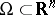with a piecewise smooth boundary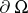. A numberis a Neumann eigenvalue ofif there exists a function(a Neumann eigenfunction) satisfying the following Neumann boundary value problem (cf. also Neumann boundary conditions):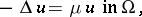(a1)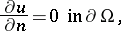(a2)

whereis the Laplace operator (i.e.,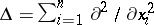). For more general definitions, see [a8]. Neumann eigenvalues (with) appear naturally when considering the vibrations of a free membrane (cf. also Natural frequencies). In fact, forthe non-zero Neumann eigenvalues are proportional to the square of the eigenfrequencies of the membrane with free boundary. Providedis bounded and the boundaryis sufficiently regular, the Neumann Laplacian has a discrete spectrum of infinitely many non-negative eigenvalues with no finite accumulation point:(a3)

(as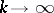). The Neumann eigenvalues are characterized by the max-min principle [a3]: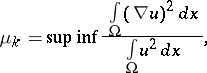(a4)

where the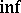is taken over all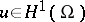orthogonal to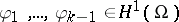, and the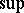is taken over all the choices of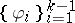. For simply-connected domains the first eigenfunction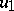, corresponding to the eigenvalue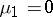is constant throughout the domain. All the other eigenvalues are positive. While Dirichlet eigenvalues satisfy stringent constraints (e.g.,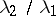cannot exceed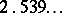for any bounded domain in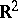, [a1]; see also Dirichlet eigenvalue), no such constraints exist for Neumann eigenvalues, other than the fact that they are non-negative. In fact, given any finite sequence, there is an open, bounded, smooth, simply-connected domain ofhaving this sequence as the firstNeumann eigenvalues of the Laplacian on that domain [a2]. Though it is obvious from the variational characterization of both Dirichlet and Neumann eigenvalues (see (a4)) that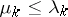, L. Friedlander [a4] proved the stronger result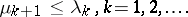(a5)

How far the first non-trivial Neumann eigenvalue is from zero for a convex domain inis given through the optimal inequality [a7]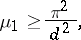(a6)

whereis the diameter of the domain. There are many more isoperimetric inequalities for Neumann eigenvalues (see Rayleigh–Faber–Krahn inequality).

For large values of, H. Weyl proved [a9](a7)

where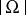and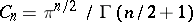are, respectively, the volumes ofand of the unit ball in.

For any plane-covering domain (i.e., a domain that can be used to tile the plane without gaps, nor overlaps, allowing rotations, translations and reflections of itself), G. Pólya [a6] proved that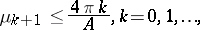(a8)

and conjectured the same bound for any bounded domain in. This is equivalent to saying that the Weyl asymptotics of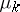is an upper bound for. The analogous conjecture in dimensionis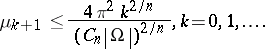(a9)

The most significant result towards the proof of Pólya's conjecture for Neumann eigenvalues is the result by P. Kröger [a5]:A proof of Pólya's conjecture for both Dirichlet and Neumann eigenvalues would imply Friedlander's result (a5).

How to Cite This Entry:
Neumann eigenvalue. Encyclopedia of Mathematics. URL: http://encyclopediaofmath.org/index.php?title=Neumann_eigenvalue&oldid=12806
This article was adapted from an original article by Rafael D. Benguria (originator), which appeared in Encyclopedia of Mathematics - ISBN 1402006098. See original article